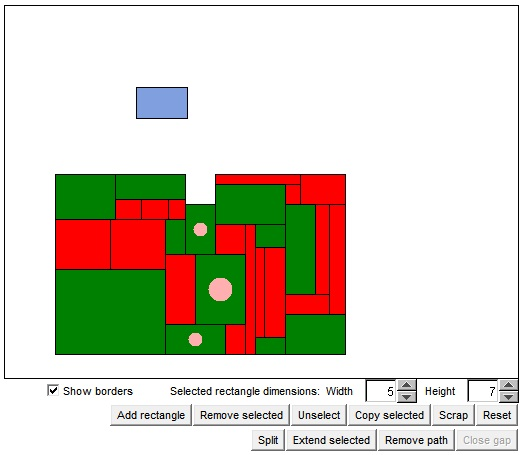# Integers and Rectangles:a Proof by Induction

A curious problem has been discussed by Stan Wagon not so long ago.

Whenever a rectangle is tiled by rectangles each of which has at least one integer side, then the ambient rectangle has at least one integer side.

In the paper, Wagon listed and discussed fourteen essentially different solutions that ranged from elementary to those that used integrals, graph theory, or topology (Sperner's lemma).

The most elementary solution was given by R. Rochberg and S. K. Stein. Another simple solution has been published by P. Winkler after Wagon's paper came out. Here, I'd like to present one other elementary proof from the paper (#9 by Raphael Robinson.)

This one is by induction.

The applet below helps with understanding this solution and even leads to another one, apparently #15.5.

Assume a big rectangle is tiled with small ones, each of which has an integer side. In the applet the ones with integer width are colored green and those with integer height red: H- and V-tiles, respectively. In case where both dimensions are integer, the color assignment is arbitrary and can be changed be clicking twice on the rectangle. (Clicking twice is not the same as a double click.)

The applet allows one to add or remove rectangles, select one at a time, and change dimensions of the selected rectangle. All rectangles are draggable.

The start up configuration is taken from Winkler's book. So, the H-tiles are green, the V-tiles are red. This is too general a configuration for Robinson's proof that deals only with H-tiles of width 1 and V-tiles of height 1. However, note that there is no loss of generality: any H-tile can be split into a number of H-tiles of unit width, and similar for V-tiles. In the applet, there is the "Split" button that does the splitting job for you.

The proof is by induction on the number of, say, H-tiles. If there is no H-tiles, the result is obvious. All the tiles are V-tiles. One can choose a sequence of V-tiles, with the first at the bottom of the ambient rectangle, and the last reaching its top edge, and those in the middle stacked on top of each other. Since each of them has an integral height, their total height is also integer.

Assume the number of H-tiles is N > 0. Choose any H-tile and call it T0. If there are H-tiles whose bottom edge abuts the top edge of T0, pick one of them and call it T1. In the absence of such H-tiles, the top edge of T0 abuts the bottom edge of one or more of the V-tiles. We then grow T0 one unit up, and, as a compensation, cut off the abutting V-tiles. This operation may increase the number of V-tiles, but not that of H-tiles.

We proceed now with either T0 or T1 as the case may be in exactly the same fashion. We stop when we reach the top of the ambient rectangle. If need be, we then apply the same procedure to T0, but now downwards.

Eventually, we shall obtain a sequence of H-tiles on top of each other, the sequence stretching from the bottom to the top of the ambient rectangle. (In the applet just press the "Extend selected" button.) The sequence can be removed (by pressing "Remove path" button.) The resulting gap between to parts of the configuration so obtained can be glued back together by shifting the lower part 1 unit up. (Use the "Close gap" button".)

The new ambient rectangle contains fewer H-tiles. In addition, if any of its sides is an integer, so will be an appropriate side of the original rectangle. Which completes the induction.

### If you are reading this, your browser is not set to run Java applets. Try IE11 or Safari and declare the site https://www.cut-the-knot.org as trusted in the Java setup.What if applet does not run?

Now, for the proof #15.5. This one is by induction on the length of the longest side of the ambient rectangle. Granted, it may not be an integer. But Robinson's operation allows one to reduce it by 1, regardless. (In the applet, Robinson's method applies to both H-tiles and, similarly, to V-tiles.) If we assume that the original rectangle has non integer sides, all successive steps will lead to a rectangle with non integer side of a smaller size, but still satisfying the conditions of the problem. Eventually, we shall be left with a rectangle both of whose sides are less than 1! But clearly such a rectangle can't be tiled by smaller rectangles with at least one side an integer. A contradiction.

### References

1. S. Wagon, Fourteen Proofs of a Result About Tiling a Rectangle, Am Math Monthly, Vol. 94, No. 7 (Aug. - Sept., 1987), 601-617.
2. P. Winkler, Mathematical Puzzles: A Connoisseur's Collection, A K Peters, 2004, pp.6-7### Integer Rectangles